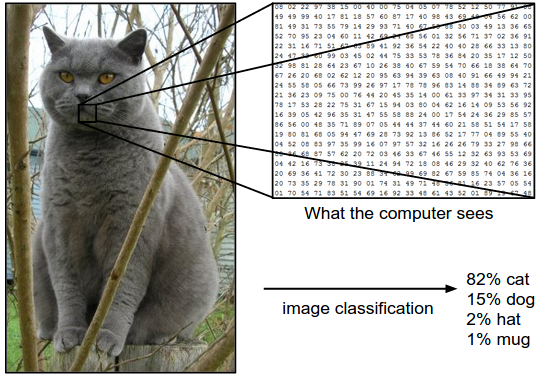## Computer vision and convolutional neural networks

Computer vision is a key aspect of artificial intelligence that is critical to many applications, from robots movements to self-driving cars and from medical imaging to products recognition in manufacturing plants. This MIT course presents the issues of computer vision and how they are handled with Convolutional Neural Networks together with the latest domains of research and state-of-the-art algorithms architectures.## Image recognition by a deep neural net

Convolution: a neuron looks for patterns in a small portion (10×10 px) of an image (256×256 px), the process is repeated by moving this small area little by litte.

Pooling: The result of the convolution is computed as a point for each portion analyzed. By a similar step by step process, a small set of points are computed into values by choosing the maximum value (“max pooling”).

By reproducing the pooling process multiple times (100x), and feeding it to a neural net, it will compute how likely the initial image is recognized as a known category.

## Autocoding

A small number of neurons (~2), the “hidden layer“, a bottleneck of neurons between two columns of multiple neurons (~10) is used to obtain output values z[n] that are the same as input values x[n].

Such results implies that a form a generalization is accomplished by the hidden layer, or rather, a form of encoded generalization, as the actual parameters of the bottleneck of neurons seems not so obvious to understand.

### Final layer of neurons

As the neural net is trained with parameters and thresholds, the shape and corresponding equation of the sigmoid function is adapted to properly sort positive and negative results, by maximizing the probability of sorting examples properly.

## Softmax

Instead of sorting by the maximum value and the corresponding category, the final output is an array of the most probable categories (~5 categories).

## Dropout

The problems of neural nets is that they can get blocked in local maximum areas. To prevent this, at each computation, one neuron is deactivated to check if its behavior is skewing the neural net. At each new computation another is shut down, or dropped out, to check all neurons.

Thanks to wider neural networks, neural nets can avoid being jammed into local maximum as they can analyze local maximum through more parameters.